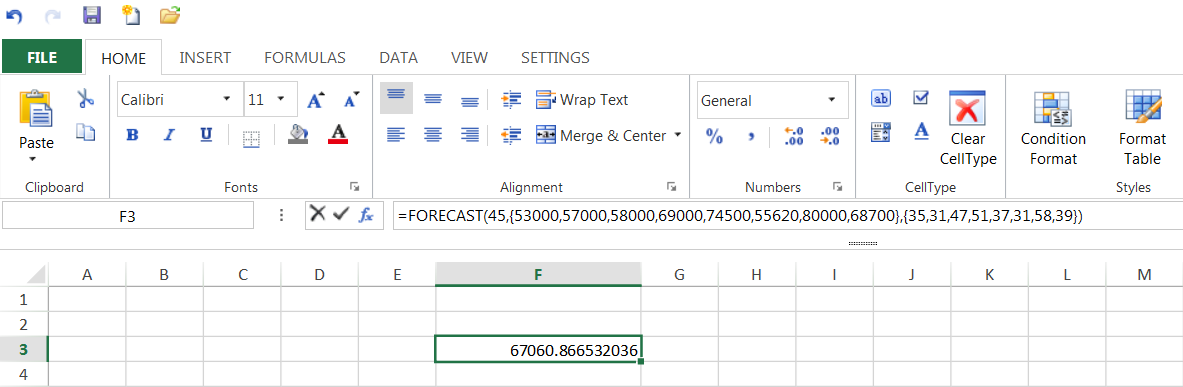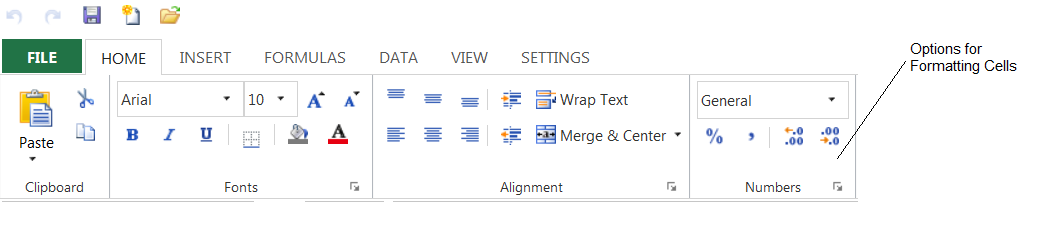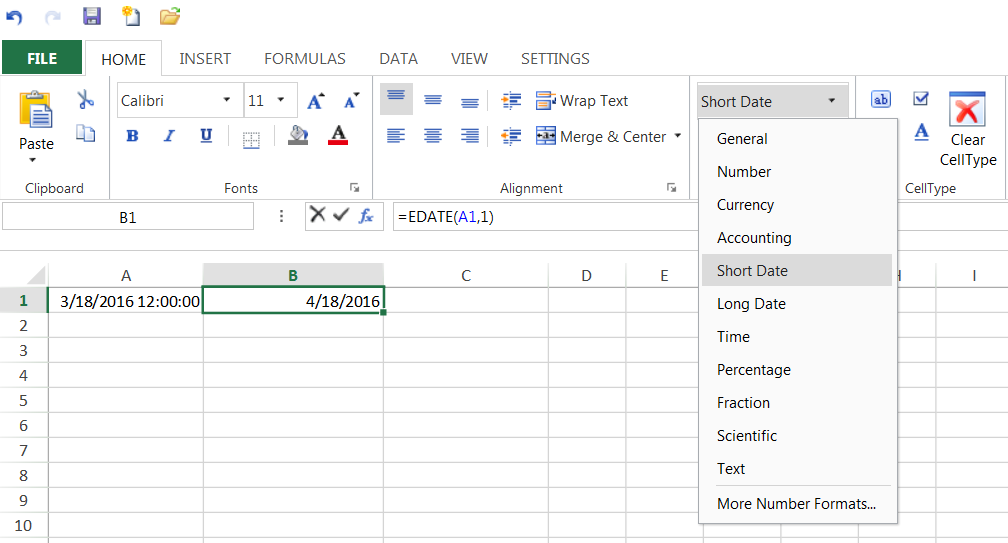﻿ SpreadJS Formulas and Cell Formatting# SpreadJS Formulas and Cell Formatting

You can use cell formatting with formulas in SpreadJS to get the results you want. Some formulas may not return the number of decimal places you want or the types of values you want to see. For example, some formulas may return more decimal places than you want to see in the cell. You can change that by setting the formatter method for the cell. This example limits the number of decimal places in the cell after using the FORECAST formula.Original Formula Result You can see the formula result in the designer as shown in the preceding image. The following code example shows how to format the result. JavaScript

``````activeSheet.setFormula(2,3,"FORECAST(45,{53000,57000,58000,69000,74500,55620,80000,68700},{35,31,47,51,37,31,58,39})",GcSpread.Sheets.SheetArea.viewport);
activeSheet.getCell(2, 3).formatter("########0");

``````

This example also sets formatting for the formula cell: JavaScript

``````activeSheet.setValue(0,0,1);
activeSheet.setValue(1,0,2);
activeSheet.setValue(2,0,3);
activeSheet.setValue(3,0,4);
activeSheet.setValue(4,0,5);
activeSheet.setValue(5,0,6);
activeSheet.setValue(6,0,7);
activeSheet.setValue(7,0,8);
activeSheet.setValue(8,0,9);

activeSheet.setValue(0,1,15.53);
activeSheet.setValue(1,1,19.99);
activeSheet.setValue(2,1,20.43);
activeSheet.setValue(3,1,21.18);
activeSheet.setValue(4,1,25.93);
activeSheet.setValue(5,1,30);
activeSheet.setValue(6,1,30);
activeSheet.setValue(7,1,34.01);
activeSheet.setValue(8,1,36.47);

activeSheet.getCell(2, 2).formatter(".#########");

``````

SpreadJS EDATE and related functions which return a date value are designed to return the date serial value in the cell (which specifies date and time). There is no built-in formatting to set the cell format to show the date format. In SpreadJS, the cell formats use the default format of the type when no cell format is applied. This results in both the date and time showing. You can apply a cell format to the cell to specify a date-only format. For example, in A1 you can enter the formula "=DATE(2016,3,18)" and SpreadJS will show "3/18/2016 12:00:00" in the cell. Then in A2 you can enter the formula "=EDATE(A1,1)" and SpreadJS will show "4/18/2016 12:00:00" (since no cell format is applied). Then you can apply a date-only format to the cell to show only the date part. For example: JavaScript

``````activeSheet.setFormula(0,0,"DATE(2016,3,18)",GcSpread.Sheets.SheetArea.viewport);
activeSheet.setFormatter(0, 1, "MM/dd/yyyy");

``````

This example uses a DATE formula, formats the result, and then uses DATEDIF on the result. JavaScript

``````activeSheet.getCell(0,0).formula("DATE(2004,2,12)");
activeSheet.setFormatter(0, 0, "MM/dd/yyyy");
activeSheet.getCell(0,1).formula("DATE(2013,2,12)");
activeSheet.setFormatter(0, 1, "MM/dd/yyyy");
activeSheet.getCell(0,2).formula("DATEDIF(A1,B1,\\"Y\\")");

``````

This example sets the format for a Time formula. JavaScript

``````var spread = new GcSpread.Sheets.Spread(\$("#ss").get(0), { sheetCount: 3 });
sheet.setFormula(0, 0, "=TIME(14,20,12");
sheet.setFormatter(0,0,"hh:mm:ss");
sheet.setFormula(1, 0, "=TIME(14,20,12");
sheet.setFormatter(1, 0, "hh:mm:ss AM/PM");
sheet.setColumnWidth(0, 200);

``````

You can also use the designer to add formulas and format cell values. The format options are located in the Home tab under the Numbers section as illustrated in the following image.Designer Options The following image displays the format options in the drop-down list.Designer Options For more information about formulas and cell formatting, refer to the following help topics:GrapeCity

GrapeCity Developer Tools